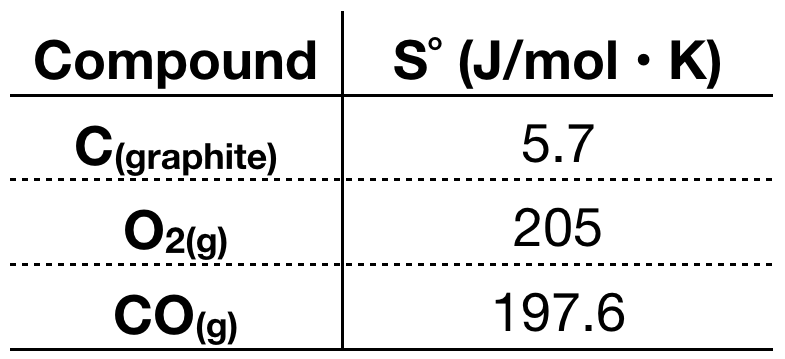# Problem: Consider the following reaction at 298 K:2C (graphite) + O2 (g) → 2CO (g)  Delta H = -110.5 kJCalculate:Delta S(sys) J/KDelta S (surr) J/KDelta S (Univ) J/K

###### FREE Expert Solution
88% (219 ratings)
###### FREE Expert Solution

#1) To calculate for ΔSsys, we need the S˚ of each compound in the reaction.88% (219 ratings)###### Problem Details

Consider the following reaction at 298 K:

2C (graphite) + O2 (g) → 2CO (g)  Delta H = -110.5 kJ

Calculate:

Delta S(sys) J/K
Delta S (surr) J/K
Delta S (Univ) J/K

What scientific concept do you need to know in order to solve this problem?

Our tutors have indicated that to solve this problem you will need to apply the Entropy concept. You can view video lessons to learn Entropy. Or if you need more Entropy practice, you can also practice Entropy practice problems.

What is the difficulty of this problem?

Our tutors rated the difficulty ofConsider the following reaction at 298 K:2C (graphite) + O2 ...as high difficulty.

How long does this problem take to solve?

Our expert Chemistry tutor, Dasha took 7 minutes and 26 seconds to solve this problem. You can follow their steps in the video explanation above.

What professor is this problem relevant for?

Based on our data, we think this problem is relevant for Professor Grant's class at HUNTER.